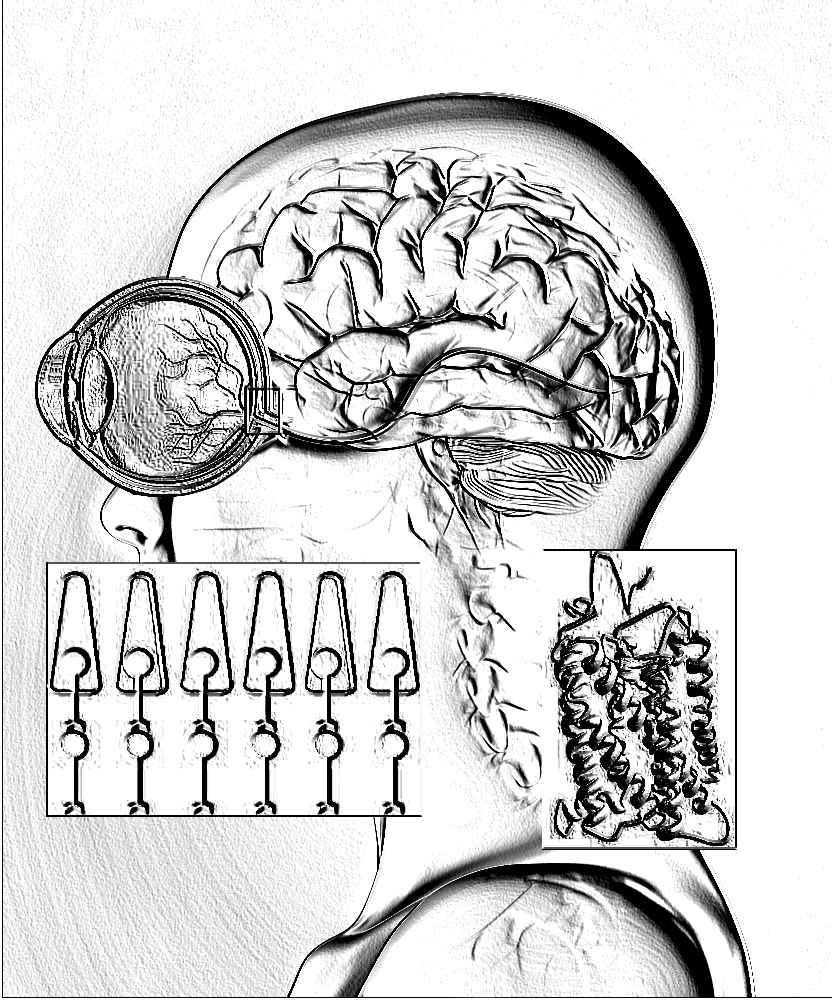## Resultats vermells

P value and statistical significance: The two-tailed P value equals 0.9281 By conventional criteria, this difference is considered to be not statistically significant. Confidence interval: The mean of Home vermell minus Dona vermell equals 0.17 95% confidence interval of this difference: From -3.62 to 3.95 Intermediate values used in calculations: t = 0.0913 df = 22 standard error of difference = 1.826 Review your data: Group Mean SD SEM Home vermell 250.33 4.64 1.34 12 Dona vermell 250.17 4.30 1.24 12

## Resultats verd

P value and statistical significance: The two-tailed P value equals 0.8779 By conventional criteria, this difference is considered to be not statistically significant. Confidence interval: The mean of Home verd minus Dona verd equals -0.25 95% confidence interval of this difference: From -3.59 to 3.09 Intermediate values used in calculations: t = 0.1554 df = 22 standard error of difference = 1.609 Review your data: Group Mean SD SEM N Home verd 250.42 4.52 1.31 12 Dona verd 250.67 3.26 0.94 12

## Resultats azul

P value and statistical significance: The two-tailed P value equals 0.5135 By conventional criteria, this difference is considered to be not statistically significant. Confidence interval: The mean of Home blau minus Dona blau equals -1.25 95% confidence interval of this difference: From -5.15 to 2.65 Intermediate values used in calculations: t = 0.6642 df = 22 standard error of difference = 1.882 Review your data: Group Mean SD SEM N Home blau 249.08 5.18 1.49 12 Dona blau 250.33 3.96 1.14 12

Test de color de Fansworth Munsell

# Test de color de Fansworth Munsell

1. Hem fet el test online de Fnasworth Munsell en aquesta pàgina web.
2. Hem analitzat els resultats de 16 alumnes: 9 noies i 7 nois. Els resultats de puntuació van de 0 a 85, que representen la quantitat de colors que 'alumne ha col·locat en la posició ccorresponent.
3. Pots veure les dades dels diferents alumnes a la seguent taula

4. Sexe Edat Puntuació
fem 16 81
fem 16 67
fem 15 70
fem 15 74
fem 15 77
fem 15 77
fem 15 67
fem 15 81
fem 17 81
masc 16 58
masc 15 76
masc 15 73
masc 16 71
masc 15 62
masc 16 72
masc 15 79

5. Hem analitzat les dades amb el test estadístic t de Student, que ens dirà si hi ha diferencies estadisticament significatives entre nois i noies, en aquesta web coneguda com t calculator quickcalcs
6. A continuació pots veure els resultats que demostren que no hi ha diferencies estadisticament significatives. Ja que són de 0,05, és a dir, estic 95% segur de que els resultats entre nois i noies són iguals.

Primer calculem la mitjana i la variabilitat dels nois i les noies. Per calcular la variabilitat emprem la desviació estàndard, que té el símbol: sigma minúscula, σ

La fòrmula de desviació estandard és:

$$\sigma= \sqrt(\sum_{i=1}^n (x_i-x)^2$$

# Student t-test

The Student t-test is a statistical hypothesis test used to determine whether two sets of data are significantly different from each other. It is used when the data samples are small (less than 30), and the variances of the two samples are not known to be equal.

## Formula

The formula for calculating the t-value for a two-sample t-test is:

$$t = \frac{\bar{X}_1 - \bar{X}_2}{s_p \sqrt{\frac{1}{n_1} + \frac{1}{n_2}}}$$

where:

• $$\bar{X}_1$$ and $$\bar{X}_2$$ are the means of the two samples
• $$s_p$$ is the pooled standard deviation of the two samples, calculated as:

$$s_p = \sqrt{\frac{(n_1 - 1)s_1^2 + (n_2 - 1)s_2^2}{n_1 + n_2 - 2}}$$

• $$n_1$$ and $$n_2$$ are the sample sizes of the two groups
• $$s_1$$ and $$s_2$$ are the standard deviations of the two samples

## Interpretation

If the calculated t-value is greater than the critical t-value (which depends on the degrees of freedom and the level of significance), we reject the null hypothesis, which states that there is no significant difference between the two sets of data.

Unpaired t test results P value and statistical significance: The two-tailed P value equals 0.1660 By conventional criteria, this difference is considered to be not statistically significant. Confidence interval: The mean of Noies minus Nois equals 4.86 95% confidence interval of this difference: From -2.27 to 11.99 Intermediate values used in calculations: t = 1.4615 df = 14 standard error of difference = 3.323 Review your data: Group Mean SD SEM N Noies 75.00 5.81 1.94 9 Nois 70.14 7.52 2.84 7

Has de comparar amb la taula de t de student oficial amb els teus resultats de la t calculada

N1+N2-2p<0.05p<0.01p<0.005p<0.001
16.31431.82163.657318.309
22.9206.9659.92522.327
32.3534.5415.84110.215
42.1323.7474.6047.173
52.0153.3654.0325.893
61.9433.1433.7075.208
71.8952.9983.4994.785
81.8602.8963.3554.501
91.8332.8213.2504.297
101.8122.7643.1694.144
111.7962.7183.1064.025
121.7822.6813.0553.930
131.7712.6503.0123.852
141.7612.6242.9773.787
151.7532.6022.9473.733
161.7462.5832.9213.686
171.7402.5672.8983.646
181.7342.5522.8783.610
191.7292.5392.8613.579
201.7252.5282.8453.552
211.7212.5182.8313.527
221.7172.5082.8193.505
231.7142.5002.8073.485
241.7112.4922.7973.467
251.7082.4852.7873.450
261.7062.4792.7793.435
271.7032.4732.7713.421
281.7012.4672.7633.408
291.6992.4622.7563.396
301.6972.4572.7503.385
401.6842.4232.7043.307
501.6762.4072.6783.261
601.6712.3902.6603.232
701.6672.3812.6483.211
801.6642.3742.6393.195
1001.6602.3642.6263.174
10001.6461.9622.5813.098
1.6452.3262.5763.090

Per últim veurem una imatge de l'ull humà on es veu la retina que conte cons que detecten el color vermell, verd i blau gràcies a unes proteïnes anomenades opsines